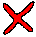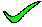The whole key s,q is not needed to determine t or u, so there are partial key dependences. Here, s->t, and q->u, so we can take t and u out of R. We must create R2 and R3 to match these two determinants, making the LHS of them keys and the RHS non-keys. However, in R2 and R3 both LHS and RHS attributes have been made keys, and this is incorrect.

closeLooking at R1, it states that s->q. There is no determinate which states this, so this option MUST be wrong.

closeThe whole key s,q is not needed to determine t or u, so there are partial key dependences. Here, s->t, and q->u, so we can take t and u out of R.

closeThis is actually true, as s,q->t and s,q->u also means s,q->t,u, which is as stated in R. However, it still is not normalised. You need to take into account the s->t and q->u determinants.

closeThis is actually a valid relation, as it is exactly what is stated in R. However, it still is not normalised. You need to take into account the s->t and q->u determinants.

close

 A B C D E TELL ME NEXT INDEX

Consider the following functional dependencies

 a,b => c,d e,g,h => f,j a,c => b,d p,q => r,s e,f,g => h,i s => t f,g => j q => u g,h => i

Which of the following relational schemas might be the result of normalising R(s,q,t,u)?
1. The schema R1(s,q) R2(s,t) R3(q,u)
2. The schema R1(s,q) R2(q,t) R3(t,u)
3. The schema R1(s,q) R2(s,t) R3(q,u)
4. The schema R1(s,q,t) R2(s,q,u)
5. The schema R(s,q,t,u)